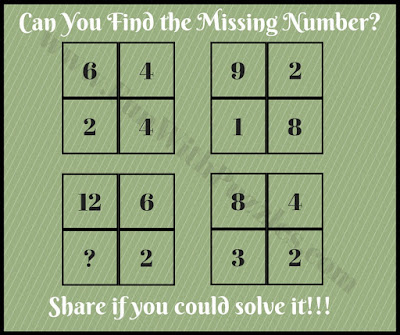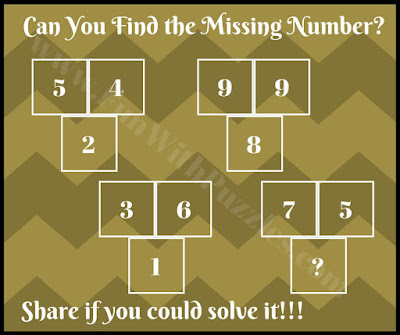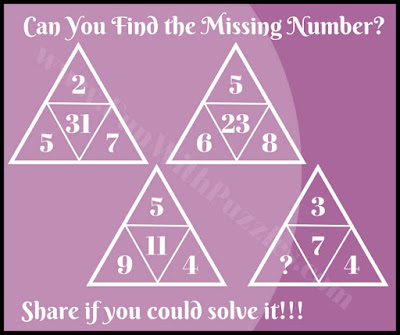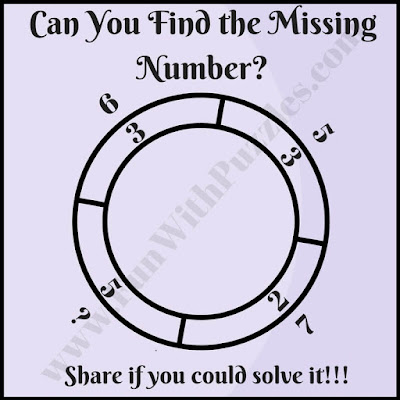## Wednesday, May 6, 2020

From the last few weeks, we have been posting the Mathematical puzzles. These mathematical puzzles were based upon some geometrical shape around which few numbers have been arranged. These numbers have some mathematical pattern or arithmetically related to each other. There where many different shapes used for these mathematical brain teasers e.g. Triangles, Squares, Star, Circle or Rectangles. It time to have now a Maths Brain Test in which your mathematical ability will be tested. This Brain Test is having some very interesting and confusing math brain puzzles. A further link is given after each puzzle for more similar puzzles. Do explore these puzzles once you have done with this test or you can first try solving these puzzles then can come back for this Maths Brain Test.
There are five Mathematical Brain Teasers in this Math Brain Test. In each of the puzzle, there is some number around a given mathematical shape. These number follows some mathematical sequence or arithmetic operations so these number related to each other. Your task is to find this relationship and then find the missing number which will replace in the question mark.
So are you ready for the Maths Brain Test? Try to finish this test within 5 minutes and post your answers in the comment section.1. Tricky math quiz picture puzzle2. Tough math puzzle to find the missing number3. Tricky math triangle IQ riddle4. Tricky crossed square math brain teaser riddle5. Tough math double circle picture puzzle question

1.1.It will be great if you can also post explanation of your answer for these puzzles :)

2.3rd answer is 6, there is relation between left upper and left bottom triangle, left upper, if you start from 7 and substract first number going counter clockwise, it's number 2, you will get number 5. Left bottom triangle has same logic but clockwise and starting from number 9, 9-5=4. Relation between right upper and bottom tringles, upper has numbers on the base line 8 i 6, so difference is 2 , same logic is applied on the bottom triangle but place of numbers are switched (6,4), upper number in both triangles is lesser for 1 or 3 than next number on the base line. If you substract middle number in the upper right triangle from same one in the upper left, you will get 8 and that number is written within upper left triangle (right on the base line), same logic is applied on the bottom triangles.

3.2. ans = 3
5*4 = 20 --> 2
9*9 = 81 --> 8
==> 7*5 = 35 --> ? = 3

3. ans = 4
5*7 - 2^2 = 31
==> ? = 4

4. ans = 28
6 + 2 + 4 + 3 = 15
6 + 6 + 9 + 4 = 25
9 + 8 + 12 + 6 = 35
==> ? = 45 - (3 + 2 + 12) = 28

5. ans = 8
5 + 2 = 7
2 + 3 = 5
3 + 3 = 6
==>? = 3 + 5 = 8

1.Sorry, ans of Question 4 is 8
6*2 = 3*4
6*6 = 4*9
9*8=6*12
==> ? = 24/3 = 8

A comment doesn't cost a thing. Please drop a comment below to boost the author's morale.